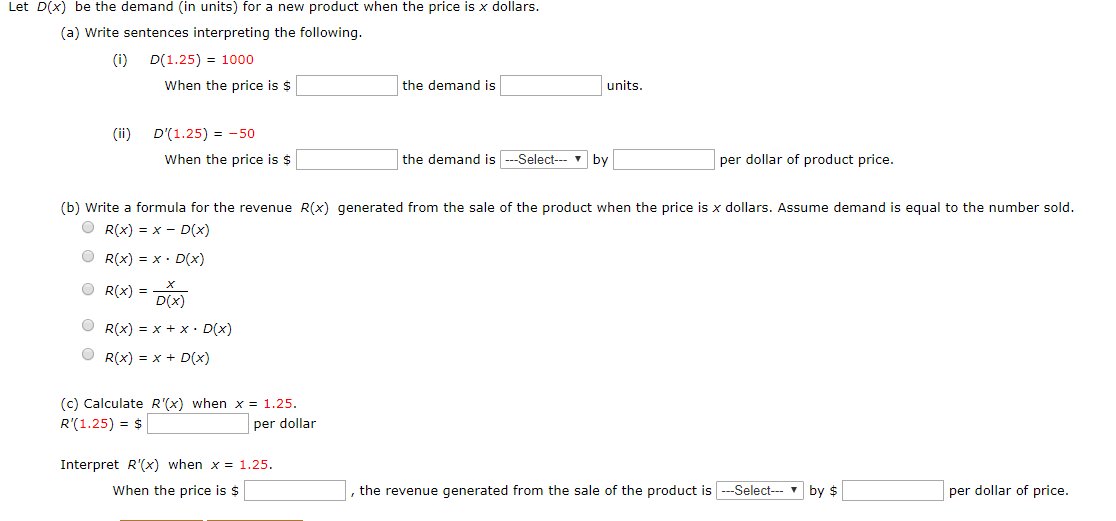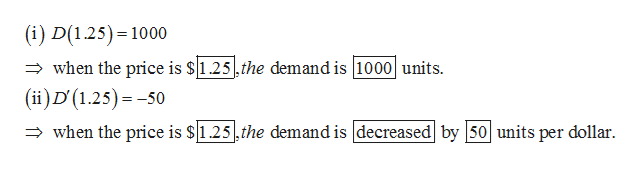# Let D(x be the demand (in units) for a new product when the price is x dollars.(a) Write sentences interpreting the following.D(1.25) 1000When the price is \$the demand isunits(ii)D'(1.25) = -50the demand is SelectWhen the price is \$per dollar of product price.by(b) Write a formula for the revenue R(x) qenerated from the sale of the product when the price is x dollars. Assume demand is equal to the number sold.OR(x) x- D(x)R(x) x D(x)OR(x)Dix)R(x) x x - D(x)R(x) xD(x)(c) Calculate R'(x) when x = 1.25.R'(1.25) \$per dollarInterpret R'(x) when x = 1.25.the revenue generated from the sale of the product isSelect- by \$When the price is \$per dollar of price.

Questionhelp_outlineImage TranscriptioncloseLet D(x be the demand (in units) for a new product when the price is x dollars. (a) Write sentences interpreting the following. D(1.25) 1000 When the price is \$ the demand is units (ii) D'(1.25) = -50 the demand is Select When the price is \$ per dollar of product price. by (b) Write a formula for the revenue R(x) qenerated from the sale of the product when the price is x dollars. Assume demand is equal to the number sold. OR(x) x- D(x) R(x) x D(x) OR(x) Dix) R(x) x x - D(x) R(x) xD(x) (c) Calculate R'(x) when x = 1.25. R'(1.25) \$ per dollar Interpret R'(x) when x = 1.25. the revenue generated from the sale of the product isSelect- by \$ When the price is \$ per dollar of price. fullscreen
check_circleExpert Solution
Step 1

(a)help_outlineImage Transcriptionclose(i) D(1.25) 1000 when the price is \$1.25,the demand is 1000 units (ii)D (1.25) -50 when the price is \$1.25,the demand is decreased by 50 units per dollar. fullscreen
Step 2

(b) The formula for the reve...

### Want to see the full answer?

See Solution

#### Want to see this answer and more?

Solutions are written by subject experts who are available 24/7. Questions are typically answered within 1 hour*

See Solution
*Response times may vary by subject and question
Tagged in

### Other